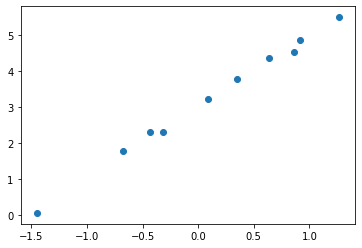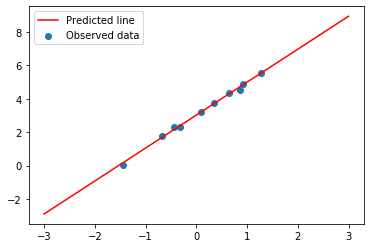In :
from sklearn.linear_model import LinearRegression
import numpy as np
import pandas as pd
import matplotlib.pyplot as plt

In :
x = np.random.randn(10,1)

## adding some noise so that it's not just a straight line
y = 2*x+3 + 0.1*np.random.randn(10,1)

plt.scatter(x,y)
plt.show()In :
x

Out:
array([[ 0.86209977],
[-0.31920793],
[ 0.63691567],
[ 0.09190243],
[ 0.35366852],
[-1.45174052],
[-0.67590895],
[ 1.26842744],
[-0.43573289],
[ 0.91497179]])
In :
model = LinearRegression()
model.fit(x,y)

Out:
LinearRegression(copy_X=True, fit_intercept=True, n_jobs=None, normalize=False)
In :
model.coef_

Out:
array([[1.97229407]])
In :
model.intercept_

Out:
array([3.02744237])
In :
model.predict([])

Out:
array([[42.47332372]])
In :
2*20+3

Out:
43
In :
## Got a whole bunch of test values in the range of -3 > x > 3
x_test = np.linspace(-3,3)

## Then I'm going to predict them. Making sure 'x_test' is 2D array
y_pred = model.predict(x_test[:,None])

plt.scatter(x,y)
plt.plot(x_test,y_pred,'r')
plt.legend(['Predicted line','Observed data'])
plt.show()